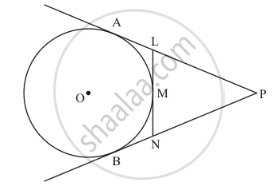# In Figure Pa and Pb Are Tangents from an External Point P to the Circle with Centre O. Ln Touches the Circle at M. Prove that Pl + Lm = Pn + Mn - Mathematics

In figure PA and PB are tangents from an external point P to the circle with centre O. LN touches the circle at M. Prove that PL + LM = PN + MN

#### SolutionGiven

O is Centre of circle

PA and PB are tangents

We know that

The tangents drawn from external point to the circle are equal in length.

From point P, PA = PB

⇒ PL + AL = PN + NB …. (i)

From point L & N, AL = LM and MN = NB } …. Substitute in (i)

PL + Lm = PN + MN

⇒ Hence proved.

Concept: Concept of Circle - Centre, Radius, Diameter, Arc, Sector, Chord, Segment, Semicircle, Circumference, Interior and Exterior, Concentric Circles
Is there an error in this question or solution?

#### APPEARS IN

RD Sharma Class 10 Maths
Chapter 8 Circles
Exercise 8.2 | Q 28 | Page 37